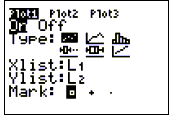# Steps for using the TI-83 or 84 for finding models for data

(Regression Models)

Enter Data (1)

> press STAT, then ENTER (in order to edit) and type ordered pairs in L1 and L2

(hint: to quickly clear an old list, go to the top and press CLEAR)

To look at a scatterplot> press 2nd STAT PLOT and make sure that a plot (probably Plot1) is set properly for a scatterplot (On, scatterplot, x-list L1, y-list L2 as shown at the right) Plot2 and Plot3 should be OFF.

> press ZOOM, 9:ZoomStat (which also sends you to GRAPH)

(> press GRAPH anytime to go to the graph)

To find the model (equation)

> press STAT, CALC

> press LinReg, QuadReg, or ExpReg... (for linear, quadratic, and exponential regression, respectively)

> press ENTER (assuming you used L1 and L2) (if you wish to use other lists, they must be specified))

Load the equation into the calculator for future use

> press Y=

(> CLEAR out the old equations--if you didn't do this initially)

> press VARS (for variables), Statistics, EQ, RegEQ (for regression equation)

To see your model graphed on top of the scatterplot

> press GRAPH (2)

To plug in an x-value into your model

> Press TRACE (press the up-arrow to trace on Y1) and type in the x-value and press ENTER (you may have to adjust the WINDOW to make the point visible).

> There are three (3) other ways to do this, including (a) TABLE; (b) 2nd|CALC|Value; (c) 2nd QUIT (to return to the home screen, if needed) |VARS (for variables), Y-Vars, Function, Y1 (or whichever function you loaded it into), "(#)" where # is a number, ENTER

Footnotes

1. Before entering data, it is good practice to press Y= and CLEAR all existing equations.

2. TRACE works nicely at this point. steps x right and left. switches from the data to the model and back.

Jim Olsen, Western Illinois University